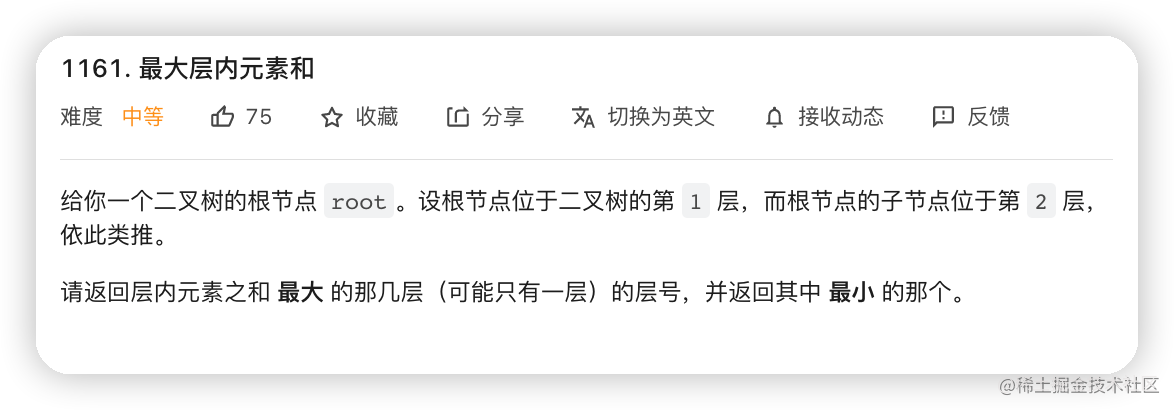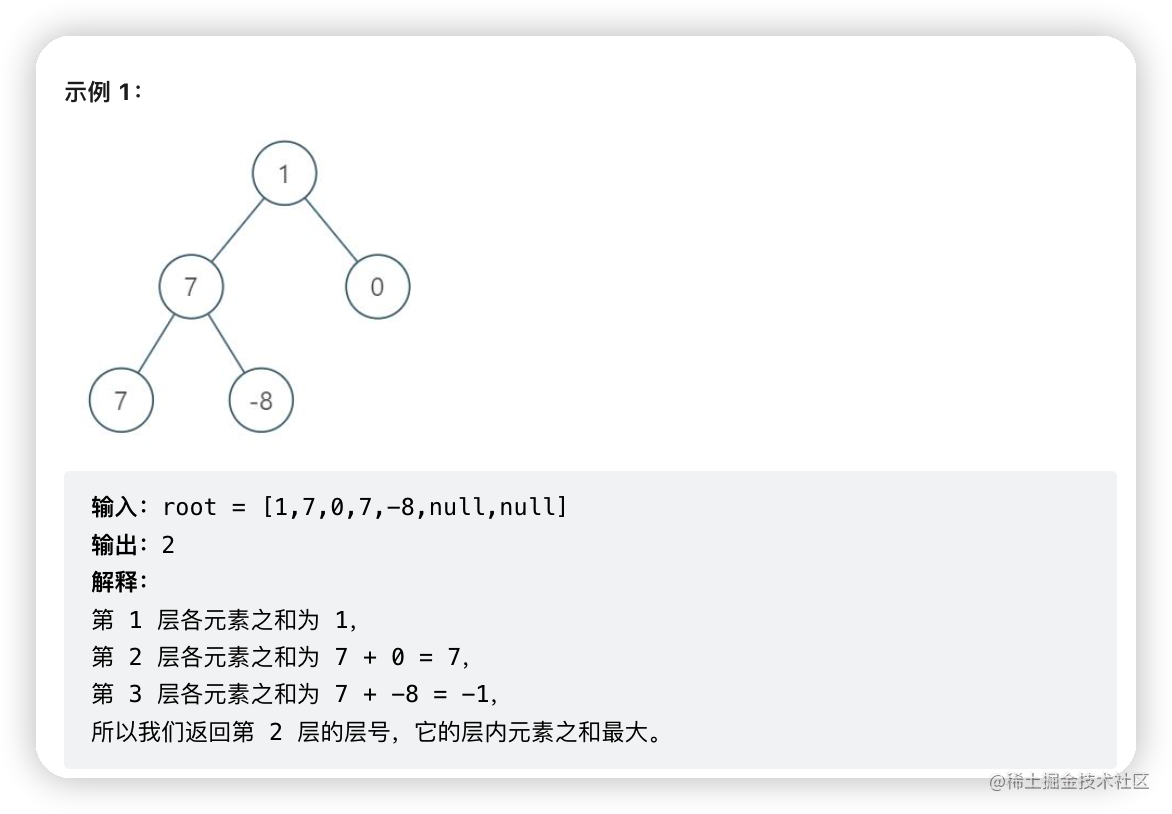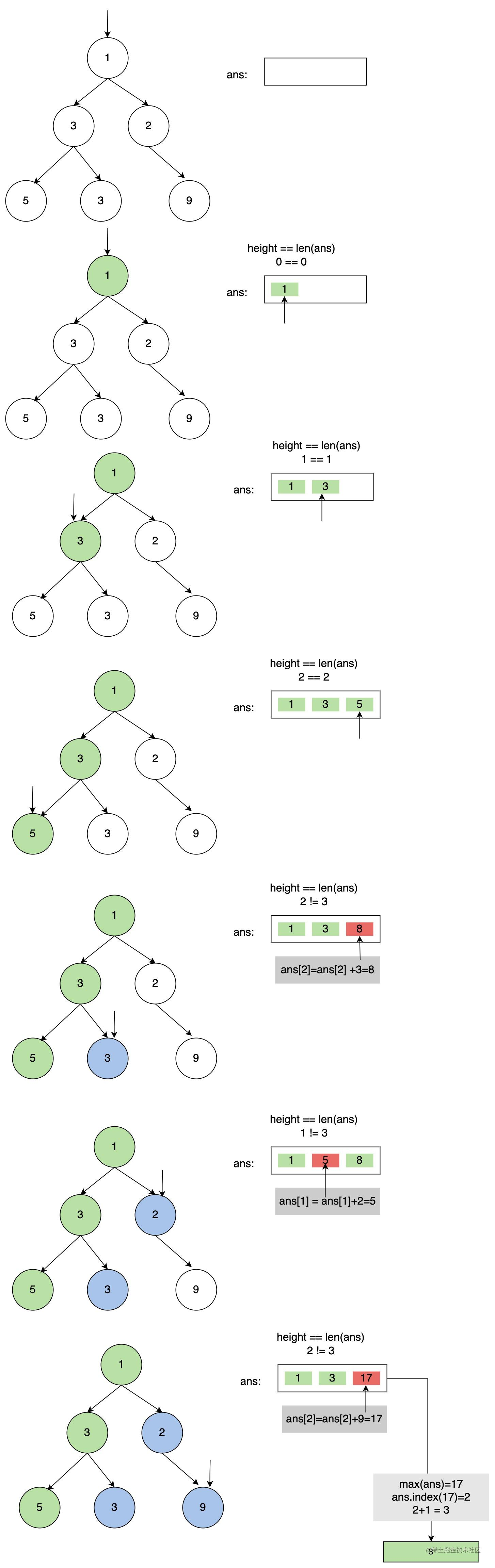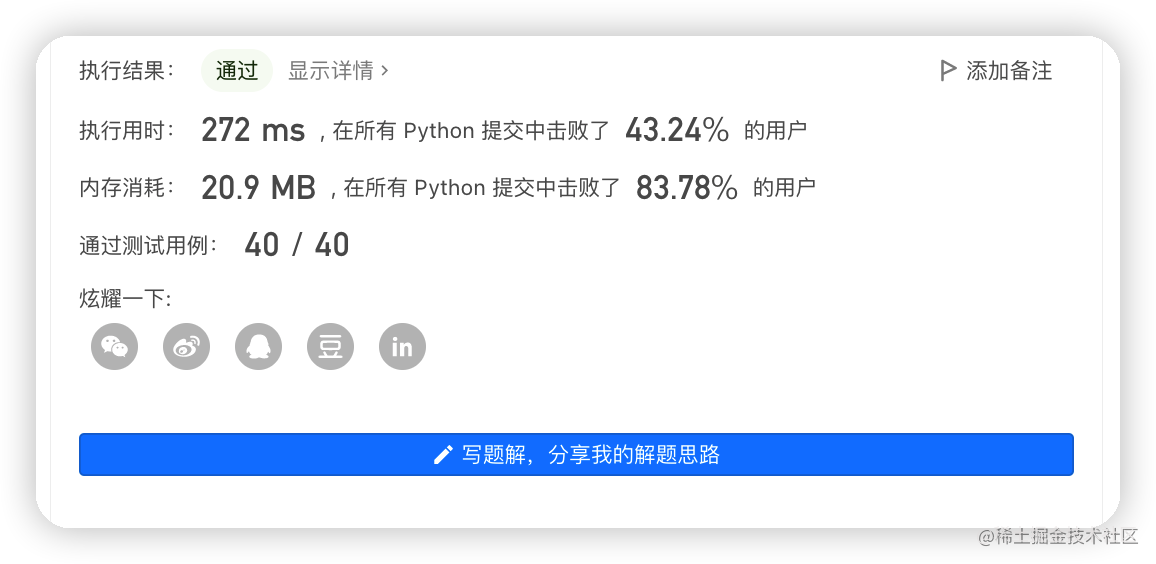## 【LeetCode】1161. 二叉树最大层内元素和# 一、题目描述：

• ## 题目内容• ## 题目示例• ## 题目解析

• 树中的节点数在 `[1, 104]`范围内
• `-105 <= Node.val <= 105`

# 二、思路分析：

• 方法一： 深度优先遍历（dfs）递归

• 使用dfs方法中常用就有前、中、后序三种遍历，本题中我们直接使用前序遍历即可
• 由于需要与二叉树的层数紧密关联的，因此我们需要定一个列表ans来存储每一层的元素和
• 列表ans的长度与二叉树的高度是一致的，通过len(ans)==height时，ans.append(root.val)
• 当len(ans)!=height时，则开始进行求和运算，覆盖原先的值
• height深度+1，继续递归添加root的左右孩子，直到root为空时，进行退出递归
• 我们通过上述思路，画图推理过程如下：根据以上思路，使用Python代码如下所示：
``````class Solution(object):
def maxLevelSum(self, root):
"""
:type root: TreeNode
:rtype: int
"""
ans = []
def dfs(root,height):
if not root:return
if len(ans) == height:
ans.append(root.val)
else:
ans[height] += root.val
height +=1
dfs(root.left,height)
dfs(root.right,height)

dfs(root,0)
return ans.index(max(ans))+1

复制代码``````
• 方法二：广度优先遍历（bfs)

• 广度优先遍历是使用队列与while结合，对二叉树每一层节点分批处理
• 定义初始化ans为1，maxSum为root.val,level值为1
• 定义一个以root节点开始的队列bfs，bfs赋值给临时数组tmp,bfs列表清空，for循环遍历tmp的所有节点值进行求和Sum,并使用清空的bfs列表添加节点的左右孩子
• 当Sum大于初始的maxSum时，则ans,maxSum重新被赋值为level，Sum
• Level深度+1，直到bfs为空为止，退出while循环``````class Solution(object):
def maxLevelSum(self, root):
"""
:type root: TreeNode
:rtype: int
"""
ans,maxSum = 1,root.val
level = 1
bfs = [root]
while bfs:
Sum = 0
tmp =bfs
bfs = []
for node in tmp:
Sum += node.val
if node.left:
bfs.append(node.left)
if node.right:
bfs.append(node.right)
if Sum > maxSum:
ans,maxSum = level,Sum
level +=1
return ans
复制代码``````

# 三、总结：• 时间复杂度：O(n),n为二叉树节点数
• 空间复杂度：O(n)，需要对二叉树节点数遍历